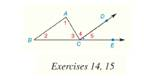Chapter 2.CT, Problem 15CT### Elementary Geometry for College St...

6th Edition
Daniel C. Alexander + 1 other
ISBN: 9781285195698

#### Solutions

Chapter
Section### Elementary Geometry for College St...

6th Edition
Daniel C. Alexander + 1 other
ISBN: 9781285195698
Textbook Problem
1 views

# In the figure shows, ∠ A C E is an exterior angle of Δ A B C . If C D → ∥ B A → , m ∠ 1 = 2 ( m ∠ 2 ) , and m ∠ A C E = 117 ° .Find measure of ∠ 1 . ______________To determine

To find:

The measure of 1.

Explanation

Given:

The following figure shows the given diagram.

CDBA, m1=2(m2), and mACE=117°.

Property used:

Measure of exterior angle of a triangle will be equal to the sum of the measure of two nonadjacent interior angles.

Calculation:

Equation for sum of three angles of triangle is as follows.

### Still sussing out bartleby?

Check out a sample textbook solution.

See a sample solution

#### The Solution to Your Study Problems

Bartleby provides explanations to thousands of textbook problems written by our experts, many with advanced degrees!

Get Started